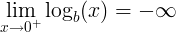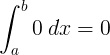# Zero number (0)

## Zero number definition

Zero is a number used in mathematics to describe no quantity or null quantity.

When there are 2 apples on the table and we take the 2 apples, we can say that there are zero apples on the table.

The zero number is not positive number and not negative number.

The zero is also a placeholder digit in other numbers (e.g: 40,103, 170).

#### Is zero a number?

Zero is a number. It is not positive nor negative number.

#### Zero digit

The zero digit is used as a placeholder when writing numbers.

#### For example:

204 = 2×100+0×10+4×1

## Zero number history

#### Who invented the zero number?

The modern 0 symbol was invented in India in the 6-th century, used later by the Persians and Arabs and later in Europe.

#### Symbol of zero

The zero number is denoted with the 0 symbol.

The Arabic numeral system uses the ٠ symbol.

## Zero number properties

x represents any number.

Operation Rule Example

x + 0 = x

3 + 0 = 3

x - 0 = x

3 - 0 = 3

x × 0 = 0

5 × 0 = 0

#### Division

0 ÷ x = 0 , when x ≠ 0

0 ÷ 5 = 0

x ÷ 0  is undefined

5 ÷ 0 is undefined

0 x = 0

05 = 0

x 0 = 1

50 = 1

0 = 0

#### Logarithm

logb(0) is undefined0! = 1

sin 0º = 0

cos 0º = 1

tan 0º = 0

0' = 0

#### Integral

∫ 0 dx = 0 + CAddition of a number plus zero is equal to the number:

x + 0 = x

5 + 0 = 5

#### Zero subtraction

Subtraction of a number minus zero is equal to the number:

x - 0 = x

5 - 0 = 5

#### Multiplication by zero

Multiplication of a number times zero is equal to zero:

x × 0 = 0

5 × 0 = 0

#### Number divided by zero

Division of a number by zero is not defined:

x ÷ 0 is undefined

#### For example:

5 ÷ 0 is undefined

#### Zero divided by a number

Division of a zero by a number is zero:

0 ÷ x = 0

0 ÷ 5 = 0

#### Number to the zero power

The power of a number raised by zero is one:

x0 = 1

50 = 1

#### Logarithm of zero

The base b logarithm of zero is undefined:

logb(0) is undefined

There is no number we can raise the base b with to get zero.

Only the limit of the base b logarithm of x, when x converges zero is minus infinity:### Sets that contain zero

Zero is an element of the natural numbers, integer numbers, real numbers and complex numbers sets:

Set Set membership notation
Natural numbers (non negative) 0 ∈ ℕ0
Integer numbers 0 ∈ ℤ
Real numbers 0 ∈ ℝ
Complex numbers 0 ∈ ℂ
Rational numbers 0 ∈ ℚ

#### Is zero even or odd number?

The set of even numbers is:

{... ,-10, -8, -6, -4, -2, 0, 2, 4, 6, 8, 10, ...}

The set of odd numbers is:

{... ,-9, -7, -5, -3, -1, 1, 3, 5, 7, 9, ...}

Zero is an integer multiple of 2:

0 × 2 = 0

Zero is a member of the even numbers set:

0 ∈ {2k, k∈ℤ}

So zero is an even number and not an odd number.

#### Is zero a natural number?

There are two definitions for the natural numbers set.

The set of non negative integers:

0 = {0,1,2,3,4,5,6,7,8,...}

The set of positive integers:

1 = {1,2,3,4,5,6,7,8,...}

Zero is a member of the set of non negative integers:

0 ∈ ℕ0

Zero is not a member of the set of positive integers:

0 ∉ ℕ1

#### Is zero a whole number?

There are three definitions for the whole numbers:

The set of integer numbers:

ℤ = {0,1,2,3,4,5,6,7,8,...}

The set of non negative integers:

0 = {0,1,2,3,4,5,6,7,8,...}

The set of positive integers:

1 = {1,2,3,4,5,6,7,8,...}

Zero is a member of the set of integer numbers and the set of non negative integers:

0 ∈ ℤ

0 ∈ ℕ0

Zero is not a member of the set of positive integers:

0 ∉ ℕ1

#### Is zero an integer number?

The set of integer numbers:

ℤ = {0,1,2,3,4,5,6,7,8,...}

Zero is a member of the set of integer numbers:

0 ∈ ℤ

So zero is an integer number.

#### Is zero a rational number?

A rational number is a number that can be expressed as the quotient of two integer numbers:

ℚ = {n/m; n,m∈ℤ}

Zero can be written as a quotient of two integer numbers.

#### For example:

0 = 0/3

So zero is a rational number.

#### Is zero a positive number?

A positive number is defined as a number that is greater than zero:

x > 0

#### For example:

5 > 0

Since zero is not greater than zero, it is not a positive number.

#### Is zero a prime number?

The number 0 is not a prime number.

Zero is not a positive number and has infinite number of divisors.

The lowest prime number is 2.

Currently, we have around 5613 calculators, conversion tables and usefull online tools and software features for students, teaching and teachers, designers and simply for everyone.